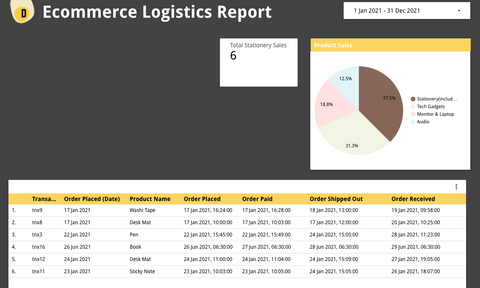# Count Distinct with Condition in Looker Studio (Formerly Google Data Studio)

Counting the total distinct numbers with defined business rules may seem simple to do in SQL, but not in Google Data Studio until I discovered this!

If you are one of the people to encounter "Can't mix metrics and dimensions" error message when trying to count distinct with a condition, I hear you.

In this post, I'd explain why you're getting such an issue and I'd share my solution with you. This article is useful for you if you want to calculate the total distinct count with one or multiple defined rules or conditions.

Love to learn from video tutorials instead? Here you go!

# Introduction with Example

Say, for example, we have a Sales datasets with the following fields:
• Transaction ID: Unique identifier for each sale transaction
• Product Name: Item name.
• Product Category: The higher category label for the product name.

If we were to calculate the total stationery orders, we have to count the total number of orders (identifiable by distinct transaction ID) with the rule of product category = "Stationery"

# First Attempt of the Combo Formula

Well, I believe many would encounter this error did the same mistake with this formula:

`IF(Category = 'Stationery',COUNT_DISTINCT(Transaction ID),0)`

Unfortunately, this is not the correct solution as we have violated the rule that metrics and dimensions cannot co-exist in the same formula.
Hence, I’m getting the error below:### So, what is the reason that the formula getting rejected?

Let’s investigate further.
• First of all, from the “surface” level, it seems that we only used 2 dimension fields: Category and Transaction ID.
• This can be further proven by the screenshot below where both fields are coloured in green. Side Note: Aggregated metrics are highlighted in blue colour instead.
• What we missed out here is the formula COUNT_DISTINCT(). This is an aggregation function that returns an aggregated metrics.
In short, if the returned result from a formula is an aggregated metric, the formula is said to have used aggregated metrics.
Therefore, it explains the error message we getting where it says we should not mix aggregated metrics with dimensions.

## How to fix this?

My solution is to use conditional formula (i.e. IF or CASE) to derive the unique transaction ID  as a new field. Then, we count the total number of unique transaction ID in the new field.

Here are 2 sample formulas:

`COUNT_DISTINCT(IF(Category="Stationery", Transaction ID,NULL))`

OR

```COUNT_DISTINCT(CASE WHEN Category="Stationery" THEN Transaction ID ELSE NULL END) ``` To explain in detail the steps involved:

1. First, we need to use IF or CASE WHEN function to define the rules.
2. Make sure that when the condition is TRUE, you returned the unique identifier as the value (In this case, the unique identifier for Sales dataset is TRANSACTION ID)
3. Return NULL when the condition is FALSE. Don’t return 0 or any other values; Otherwise, the distinct count would be inaccurate.
4. Wrap the  IF or CASE WHEN statement with COUNT_DISTINCT().Dashboard shows that total sales for stationery is at 6, which is the consistent with what we get from the raw transaction table. The above Dashboard screenshot clearly shows that total sales for stationery (as shown in a scorecard) is at 6, which is consistent with what we get from the raw transaction table.

# Alternative Solution

Other than the calculated field method in this article, we can actually use filter and scorecard to achieve the same result.
In this short 3 min video below, I covered how you could calculate and show average duration in scorecards using 2 different ways, one of it is by using a filter control within the chart. If you want to explore and understand more, click the video below to learn!